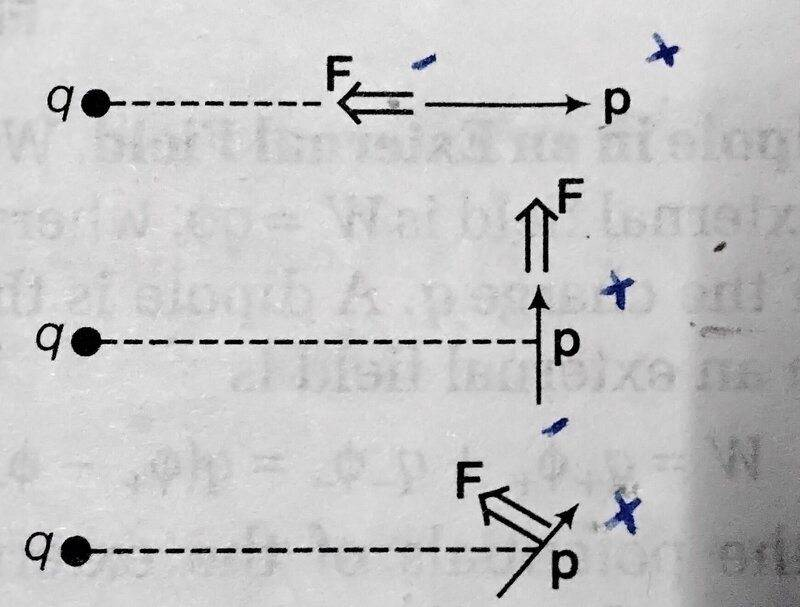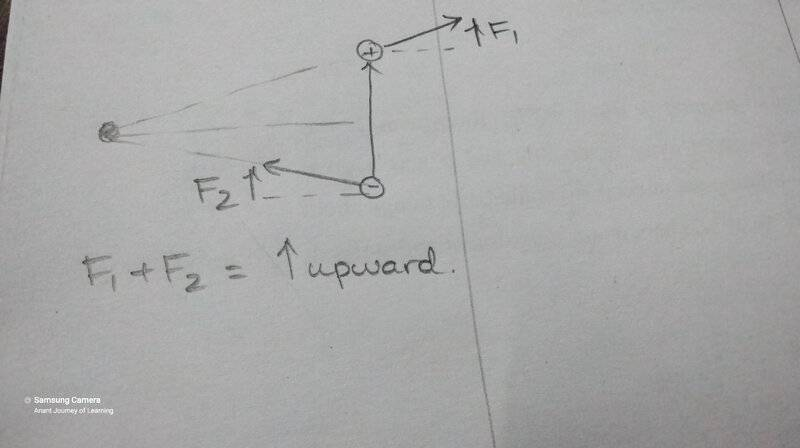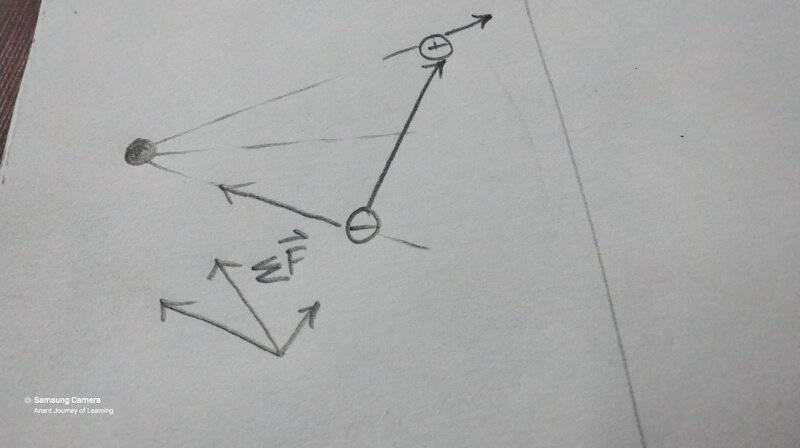# Force on a dipole in a non uniform electric field

• Shreya

#### Shreya

Homework Statement
Prove that the direction of the force on the dipole is as indicated in the diagram
Relevant Equations
F = partial derivative of p* E
I have figured out how the force is towards left in the first case. I think it is due to the larger force on - charge.Last edited:
•Delta2

Something looks off (i.e., "wrong") with the problem statement. I'm hoping your instructor didn't give you a bad problem, but it's not unheard of. Then again maybe I'm missing something myself.

From what I can tell, middle and bottom parts look wrong, although maybe the middle part is a trick question.

You haven't shown any work so we're not allowed to help help you until you do. Allow me to correct your statement,

Relevant Equations:: F=p*(partial derivative of E with respect to direction of p )

That's not quite right. The partial derivative is not necessarily in respect to the direction of $\vec p$, but rather with respect to the spatial coordinates. $\vec F$, $\vec p$, and $\vec E$ are all vectors, and they should be all subject to the chosen coordinate system.

It's true that
$$\vec F = \left( \vec p \cdot \vec \nabla \right) \vec E$$

and in Cartesian coordinates this becomes,
$$\vec F = \left( p_x \frac{\partial}{\partial x} + p_y \frac{\partial}{\partial y} + p_z \frac{\partial}{\partial z} \right) \vec E$$

but that's still not enough to reconcile the middle and bottom parts, unless maybe I'm missing something.

• Is the dipole in question just an electric dipole (as opposed to a magnetic dipole), and nothing is presently moving?
• Are all three parts intended to be taken separately (i.e., not all charges are all present simultaneously)?
• Is there anything else in this problem that wasn't stated in the problem statement, such as other constraints or other charges or fields around?
• Can you use my equation in Cartesian coordinates (above) to show that there is something wrong with the middle and bottom figures?
[Edit: Nevermind. I was misinterpreting the problem statement. The diagrams are fine.]

Last edited:
••Delta2 and Shreya
You haven't shown any work so we're not allowed to help help you until you do.
For the first case, the attractive force on the negative charge is greater than the repulsion on the positive.
So net force is towards left.

For the first case, the attractive force on the negative charge is greater than the repulsion on the positive.
So net force is towards left.
Right. I'll agree with that.

But I don't think the middle figure (diagram) and the bottom figure (diagram) are correct. [Edit: Nevermind.]

•Shreya
Is the dipole in question just an electric dipole
Yes, that's what I have understood.
Are all three parts intended to be taken separately (i.e., not all charges are all present simultaneously)?
The question is to find the net force on the dipole due to the positive charge q (on the left).
They are not separate systems

Can you use my equation in Cartesian coordinates (above) to show that there is something wrong with the middle and bottom figures?
I don't know where to start.

I think the drawings are correct. There are three ways to see this
1. Work out the formula explicitly
2. Consider the dipole as two point charges
3. Consider the force on the point charge from the dipole field (equal and opposite Newton pair) if you can picture the dipole field
For the OP: do all three!

••collinsmark and Shreya
Right. I'll agree with that.

But I don't think the middle figure and the bottom figure are correct.
For the second case, can you please check if my reasoning is right?•hutchphd and collinsmark
For the second case, can you please check if my reasoning is right?
View attachment 286528
I have not consider the perpendicular forces, since they cause a torque and not a field.

•hutchphd
But I don't think the middle figure (diagram) and the bottom figure (diagram) are correct.
It's from the book titled "Basic Laws of Electromagnetism" by IE Irodov

I think the drawings are correct. There are three ways to see this
1. Work out the formula explicitly
2. Consider the dipole as two point charges
3. Consider the force on the point charge from the dipole field (equal and opposite Newton pair) if you can picture the dipole field
For the OP: do all three!
Yes, you are correct. I was misinterpreting the problem statement, after all. Sorry about that.

For the second case, can you please check if my reasoning is right?
View attachment 286528

That's right right idea.••hutchphd and Shreya
The partial derivative is not necessarily in respect to the direction of p→, but rather with respect to the spatial coordinates
So, the partial derivative must be taken with x, y and z?
I am not very comfortable with partial derivative , so I confused myself

Will this work in the 3rd case?•collinsmark
So, the partial derivative must be taken with x, y and z?
I am not very comfortable with partial derivative , so I confused myself
You ultimately get to choose the coordinate system. So if you choose the coordinate system such that it always lines up (for example the x-axis) with the direction of $\vec p$ then you initial statement was correct.

Being able to use other coordinate systems is more flexible though.

For example in the future, you might have a system with two dipoles in different directions. So for at least one of the dipoles its direction won't line up so nicely.

•Shreya
You ultimately get to choose the coordinate system. So if you choose the coordinate system such that it always lines up (for example the x-axis) with the direction of p→ then you initial statement was correct.
Thanks a lot, collinsmark!

•collinsmark
Thank you Collinsmark and Hutchphd for helping me through this problem!•hutchphd and collinsmark